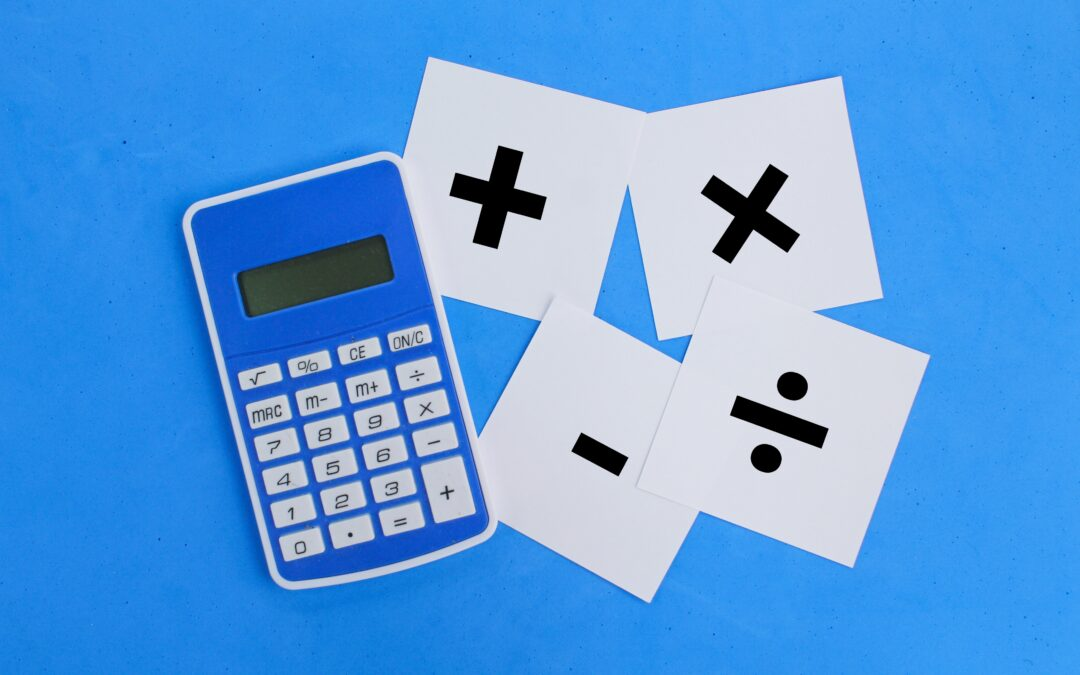Select Page## One On One Tutoring | 6 Ways to Find the Right Tutor

One on one tutoring may seem enticing to some, but chances are you may have hesitations about it actually being worth it. Many students claim they have bad experiences with tutoring but maybe they just had a bad tutor. When selecting a tutor, it is integral to choose...## What Does a Tutor Do?

What does a tutor do exactly? I’m sure this is a question you have asked yourself, whether as a student or a parent. Tutors have many different specialties. Whether you’re looking for a tutor that specializes in one subject or one that specializes in...## How Much is a Tutor?

As a parent, it can be overwhelming at the choices you have when it comes to tutors. How much is a tutor? Should I do in person or remote tutoring? Does it actually work? These are all valid questions to be asking yourself while considering a tutor for your student....## What is Tutoring?

So what is tutoring exactly? Here at TenneyTutors we pride ourselves on our one-to-one, online style of tutoring. Every single one of our tutors are one-to-one specialized private school teachers at our school: The Tenney School. The benefit of having a private school...## What Does Quotient Mean in Math?

Mathematics has a number of specified terminology that is used to describe certain types of numbers, equations, formulas, and more. So what does quotient mean in math? Well the quotient is the answer or result of dividing two numbers by each other. Lets look at some...## What Does Difference Mean in Math?

Mathematics can become quickly confusing as new concepts are introduced as students progress through higher and higher math courses. So what does difference mean in math? Well to put it shortly, the difference is the result of subtracting two or more separate numbers...